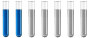## Electrolysis-Help Solve Global Warming If You Can Answer!

Chemistry and homework help forum.

Organic Chemistry, Analytical Chemistry, Biochemistry, Physical Chemistry, Computational Chemistry, Theoretical Chemistry, High School Chemistry, Colledge Chemistry and University Chemistry Forum.

Share your chemistry ideas, discuss chemical problems, ask for help with scientific chemistry questions, inspire others by your chemistry vision!

Please feel free to start a scientific chemistry discussion here!

Discuss chemistry homework problems with experts!

Ask for help with chemical questions and help others with your chemistry knowledge!

Moderators: expert, ChenBeier, Xen

QAlphaX
Jr. MemberPosts: 5
Joined: Sat Nov 13, 2021 11:07 am

### Electrolysis-Help Solve Global Warming If You Can Answer!

Here's the issue...I'm very rusty on my highschool and college chem. I came up with a great idea, but need some things answered to make sure it would work.

So, here's the problem, question and the parts needed to be answered.

Problem: I want to use hydro electric to power a electrolysis process of sea water.

Questions:
1. How much energy is needed to electrolysis any given volume of sea water?
2. What cathode and anode would be most efficient at electrolysis of sea water?
3. How much would you get of hydrogen and oxygen at any given volume of sea water @ given energy rate?
4. How much byproduct of unwanted gas is generated?
5. How much has the anode and cathode degraded over any given volume of sea water?
6. What factors may increase rate of electrolysis of same volume of water at same given rate of energy?

Answer what you can! Anything is appreciated. Leave evidence as to how you came to your answers and thx!
ChenBeier
Distinguished MemberPosts: 970
Joined: Wed Sep 27, 2017 7:25 am
Location: Berlin, Germany

### Re: Electrolysis-Help Solve Global Warming If You Can Answer!

If you use seawater which contains NaCl you will create Chlorine Gas.
The other things can be calculated. 1mol H2 need two electrons.
E = U*I*t = U*Q
QAlphaX
Jr. MemberPosts: 5
Joined: Sat Nov 13, 2021 11:07 am

### Re: Electrolysis-Help Solve Global Warming If You Can Answer!

ChenBeier wrote: Sat Nov 13, 2021 12:02 pm If you use seawater which contains NaCl you will create Chlorine Gas.
The other things can be calculated. 1mol H2 need two electrons.
E = U*I*t = U*Q
I did assume NaCl would be a byproduct.
I am hoping to understand how much on average would be produced given a specific volume of water and a specific rate of energy.
QAlphaX
Jr. MemberPosts: 5
Joined: Sat Nov 13, 2021 11:07 am

### Re: Electrolysis-Help Solve Global Warming If You Can Answer!

Here would be a given example.

If I used 1 kw/h on a 100 liters of sea water, how long will it take for the 100 liters to be used and does surface area of cathode and anode end points in the water, distance between endpoints, or other factors play an efficiency role?
ChenBeier
Distinguished MemberPosts: 970
Joined: Wed Sep 27, 2017 7:25 am
Location: Berlin, Germany

### Re: Electrolysis-Help Solve Global Warming If You Can Answer!

NaCl ist not a product its an educt. It is already present. In average the contant is 35 g/l. Its a lot.
100 l =100 kg = 5,55 kmol H2O = 11,11 kg H2
c = 0,0376 g/Ah for H+ or 0,0188 g/Ah for H2
Q = 11111g/0,0188 g/Ah = 590957 Ah
For 10 V it is 5909570 Wh = 5909,57 kWh
1 KWh = 0,00188 kg = 1,88 g
QAlphaX
Jr. MemberPosts: 5
Joined: Sat Nov 13, 2021 11:07 am

### Re: Electrolysis-Help Solve Global Warming If You Can Answer!

ChenBeier wrote: Sat Nov 13, 2021 3:15 pm NaCl ist not a product its an educt. It is already present. In average the contant is 35 g/l. Its a lot.
100 l =100 kg = 5,55 kmol H2O = 11,11 kg H2
c = 0,0376 g/Ah for H+ or 0,0188 g/Ah for H2
Q = 11111g/0,0188 g/Ah = 590957 Ah
For 10 V it is 5909570 Wh = 5909,57 kWh
1 KWh = 0,00188 kg = 1,88 g
What is g/Ah?
What is 1KWh producing 1.88g of?
ChenBeier
Distinguished MemberPosts: 970
Joined: Wed Sep 27, 2017 7:25 am
Location: Berlin, Germany

### Re: Electrolysis-Help Solve Global Warming If You Can Answer!

c = is the electrochemical equivalent the unit is g/Ah
https://second.wiki/wiki/elektrochemisc ... 4quivalent

1,88 g hydrogen
QAlphaX
Jr. MemberPosts: 5
Joined: Sat Nov 13, 2021 11:07 am

### Re: Electrolysis-Help Solve Global Warming If You Can Answer!

ChenBeier wrote: Sat Nov 13, 2021 3:15 pm NaCl ist not a product its an educt. It is already present. In average the contant is 35 g/l. Its a lot.
100 l =100 kg = 5,55 kmol H2O = 11,11 kg H2
c = 0,0376 g/Ah for H+ or 0,0188 g/Ah for H2
Q = 11111g/0,0188 g/Ah = 590957 Ah
For 10 V it is 5909570 Wh = 5909,57 kWh
1 KWh = 0,00188 kg = 1,88 g
so the 100 L is converted into 5.55 Kmol of h20? Then turned into Kg or just h2? Something seems off with these conversions. How are you going from 5.55 kmol to 11.11 kg h2?
ChenBeier
Distinguished MemberPosts: 970
Joined: Wed Sep 27, 2017 7:25 am
Location: Berlin, Germany

### Re: Electrolysis-Help Solve Global Warming If You Can Answer!

5,55 kmol H2O = 5,55 kmol H2 . The molar mass of H2 is 2g/mol or 2kg/ kmol so it will be 11,11 kg H2